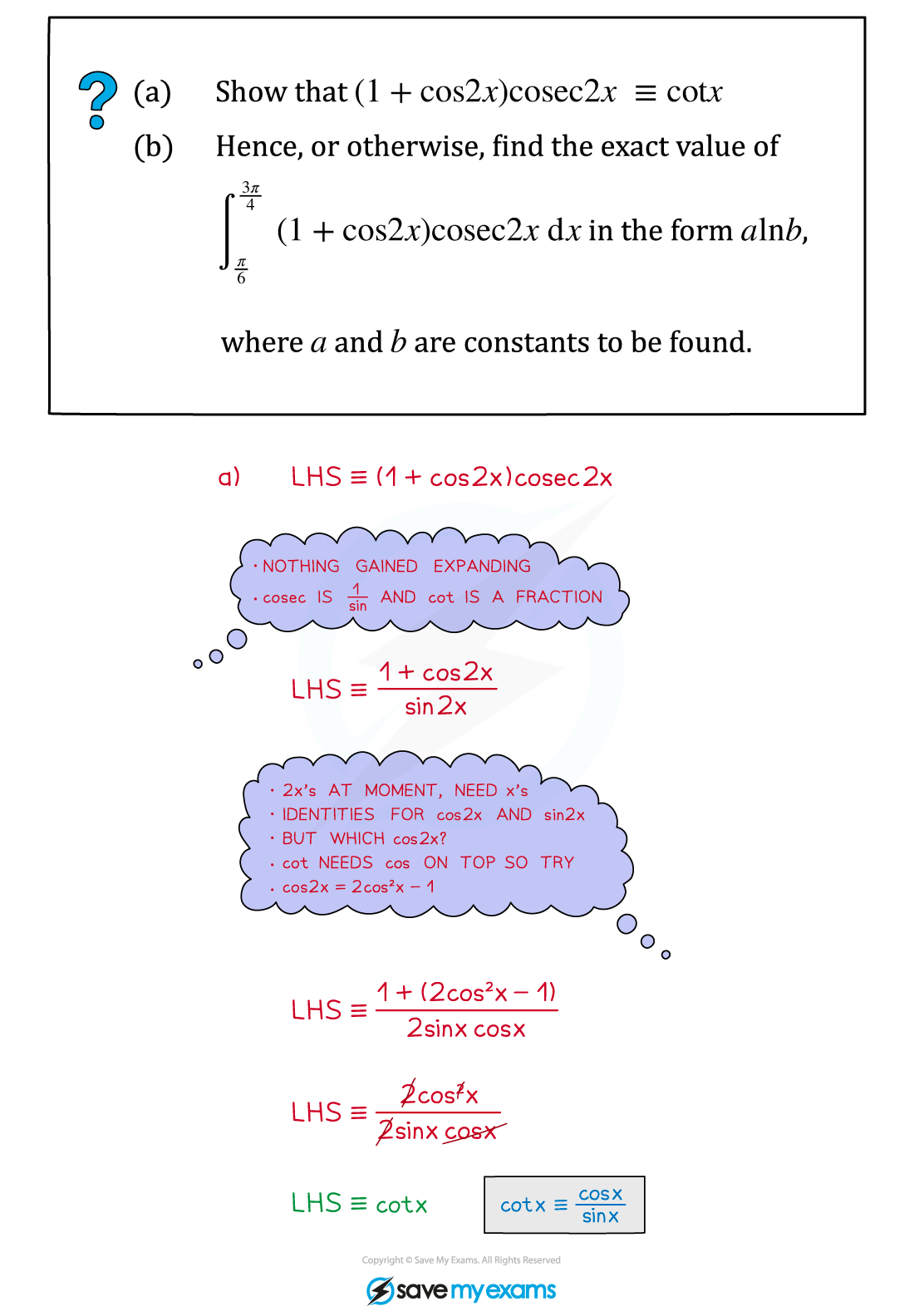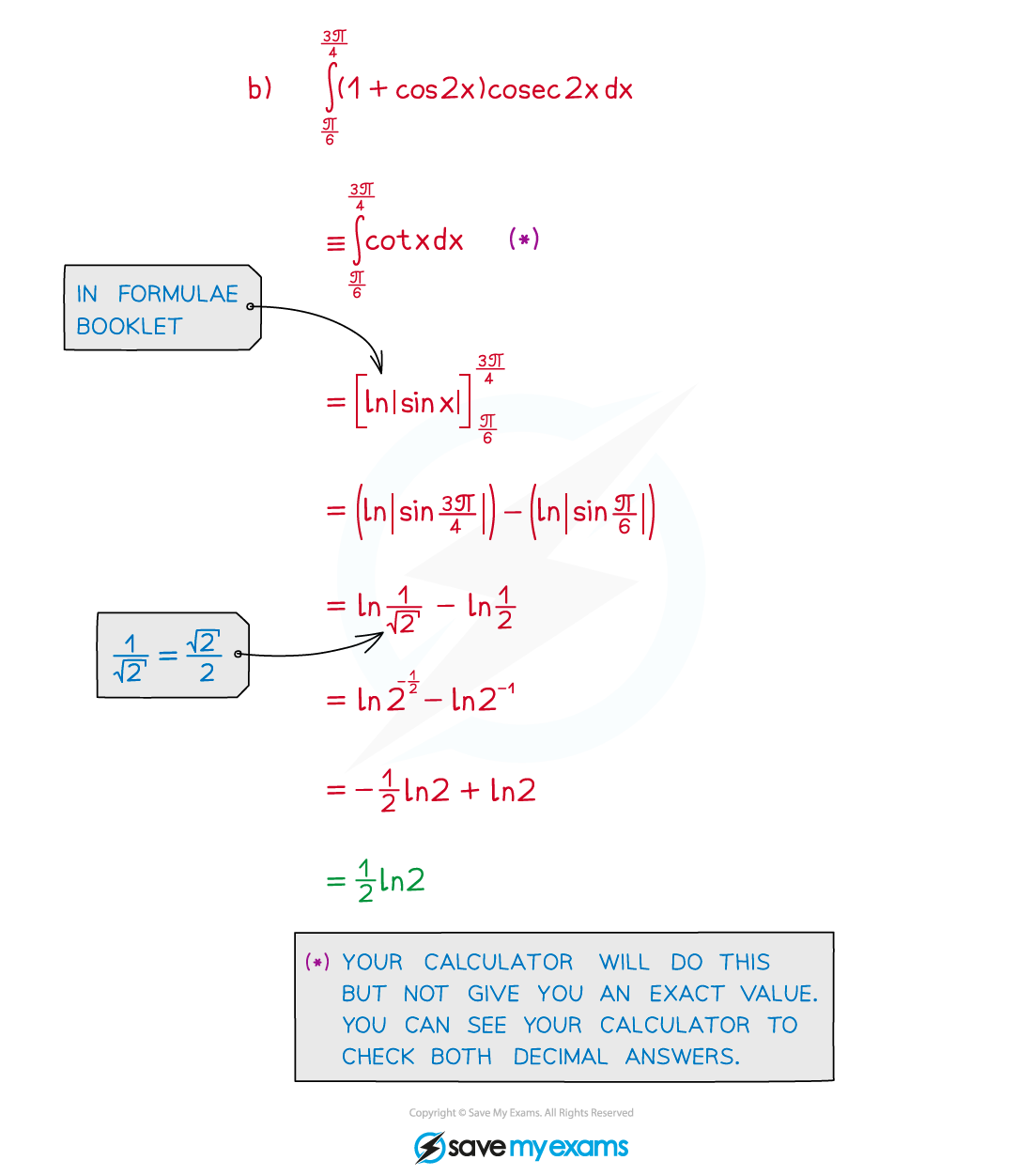# AQA A Level Maths: Pure复习笔记8.2.7 Integrating with Trigonometric Identities

### Integrating with Trigonometric Identities

#### What are trigonometric identities?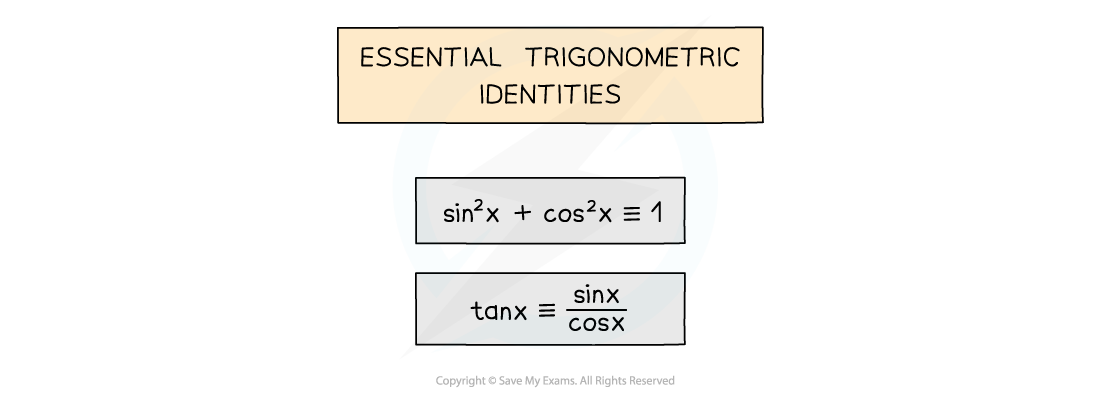• Some are given in the formulae booklet
• Be sure to note the difference between the ± and ∓ symbols!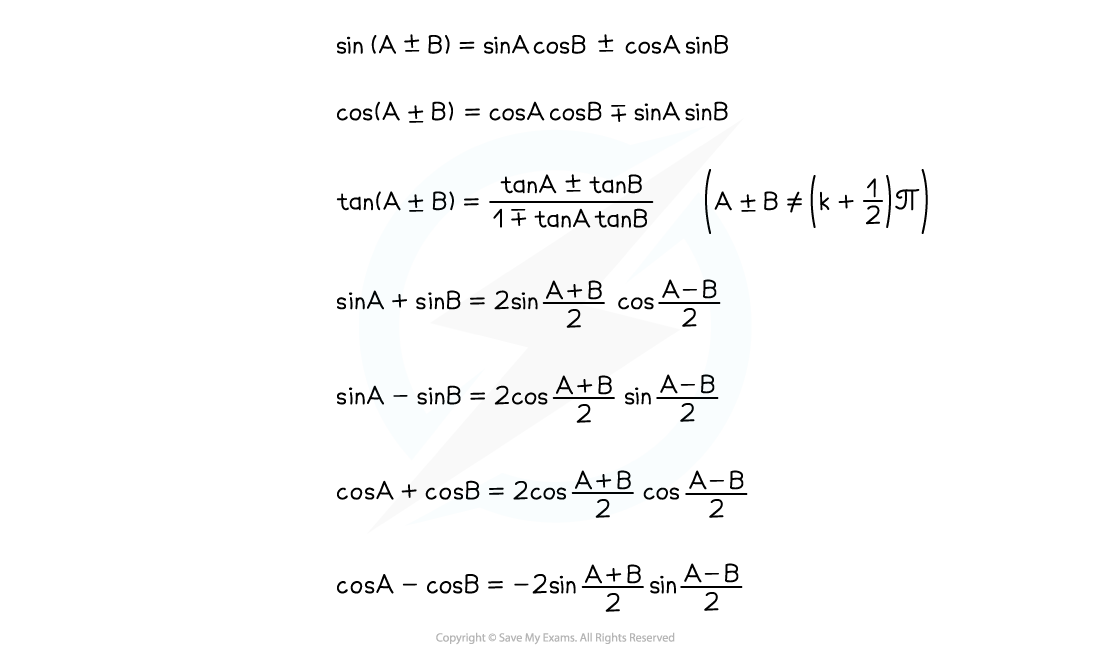#### How do I know which trig identities to use?

• There is no set method
• This is a matter of experience, familiarity and recognition
• Practice as many questions as possible
• Be familiar with trigonometric functions that can be integrated easily
• Be familiar with common identities – especially squared terms
• sin2 x, cos2 x, tan2 x, cosec2 x, sec2 x, tanx all appear in identities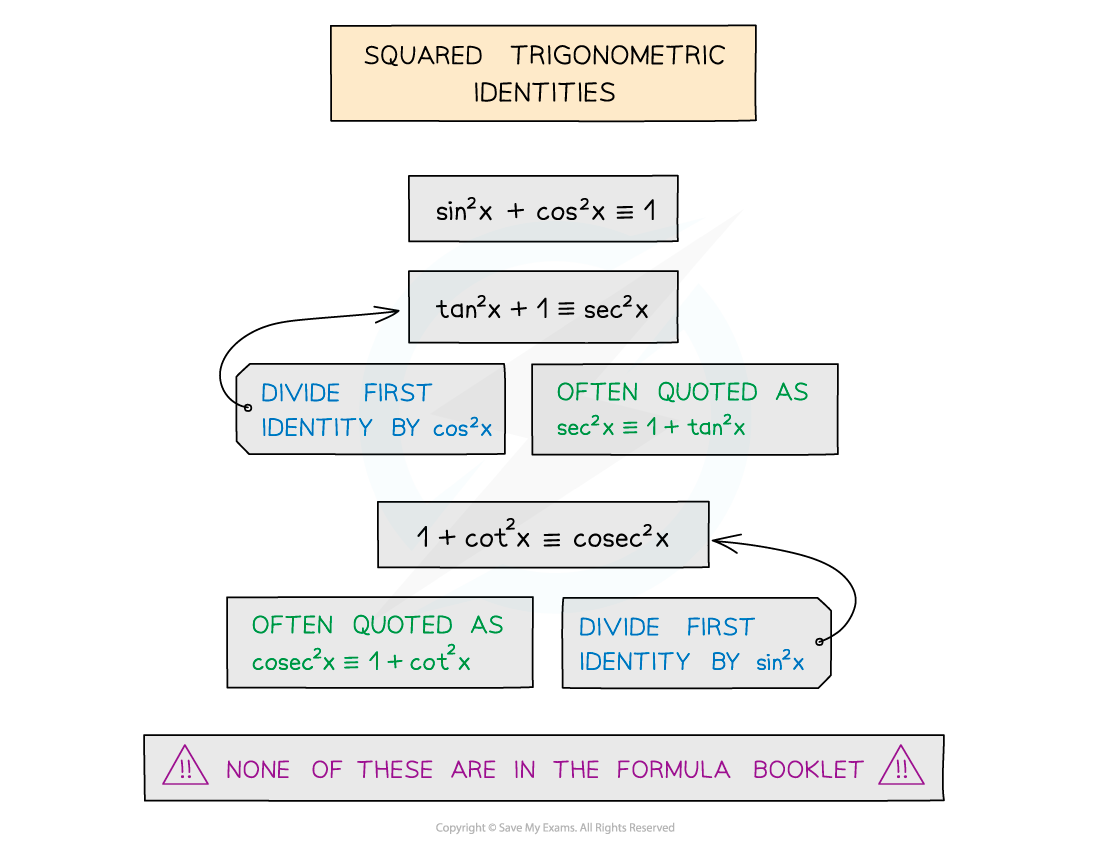#### How do I integrate tan2, cot2, sec2 and cosec2?

• The integral of sec2x is tan x (+c)
• This is because the derivative of tan x is sec2x
• The integral of cosec2x is -cot x (+c)
• This is because the derivative of cot x is -cosec2x
• The integral of tan2x can be found by using the identity to rewrite tan2x before integrating:
• 1 + tan2x = sec2x
• The integral of cot2x can be found by using the identity to rewrite cot2x before integrating:
• 1 + cot2x = cosec2x

#### How do I integrate sin and cos?

• For functions of the form sin kx, cos kx … see Integrating Other Functions
• sin kx × cos kx can be integrated using the identity for sin 2A
• sin 2A = 2sinAcosA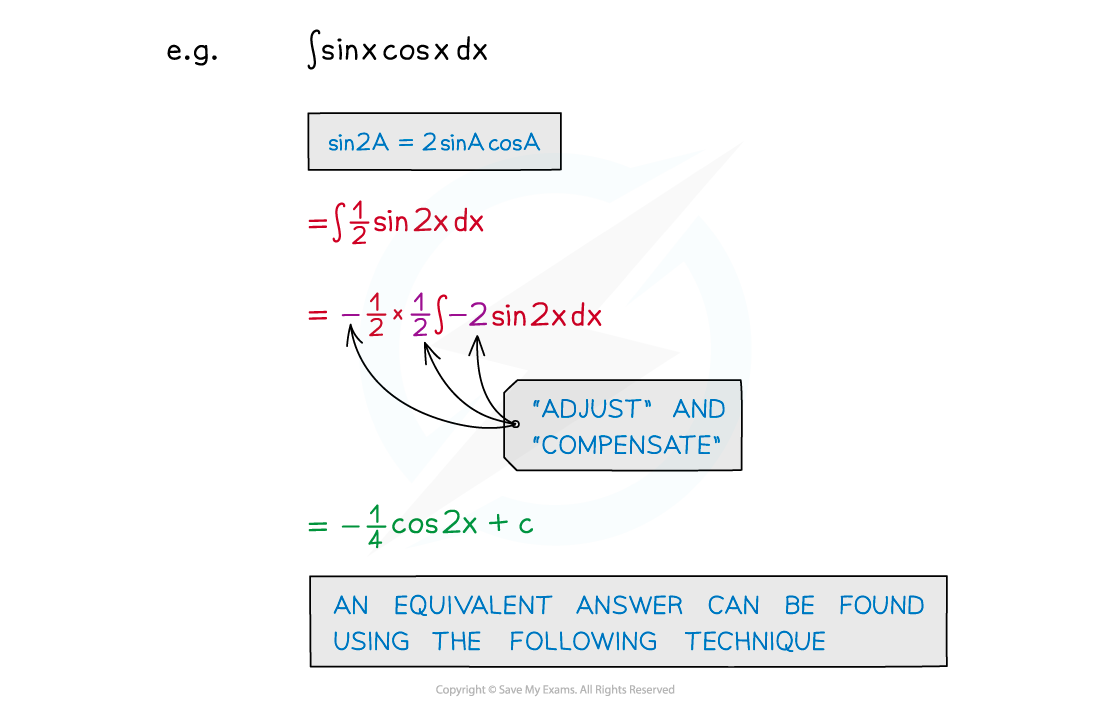• sinn kx cos kx or sin kx cosn kx can be integrated using reverse chain rule or substitution
• Notice no identity is used here but it looks as though there should be!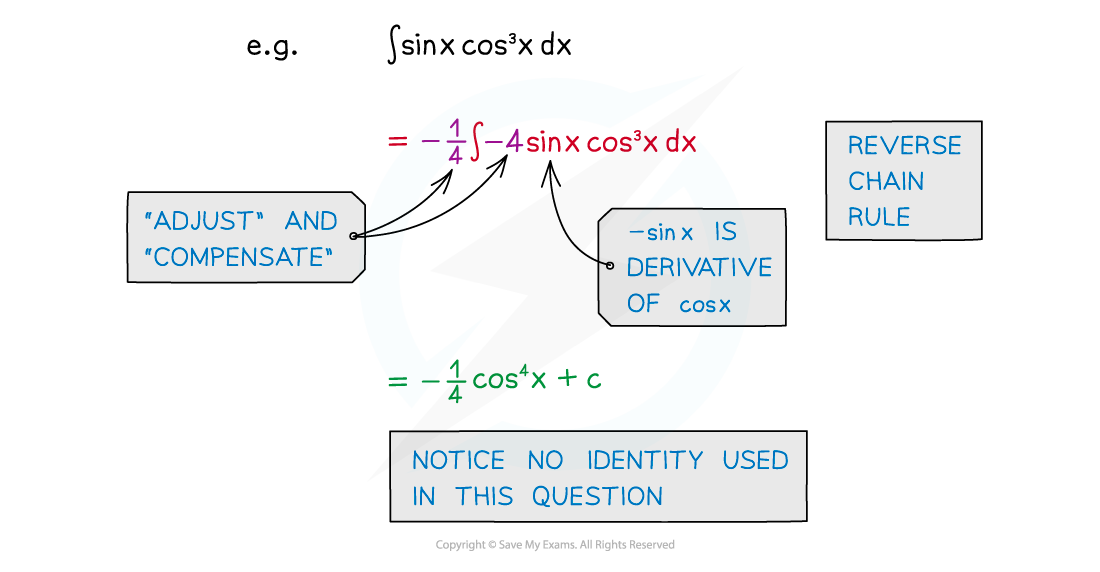• sinkx and cos2 kx can be integrated by using the identity for cos 2A
• For sin2 A, cos 2A = 1 - 2sin2 A
• For cos2 A, cos 2A = 2cos2 A – 1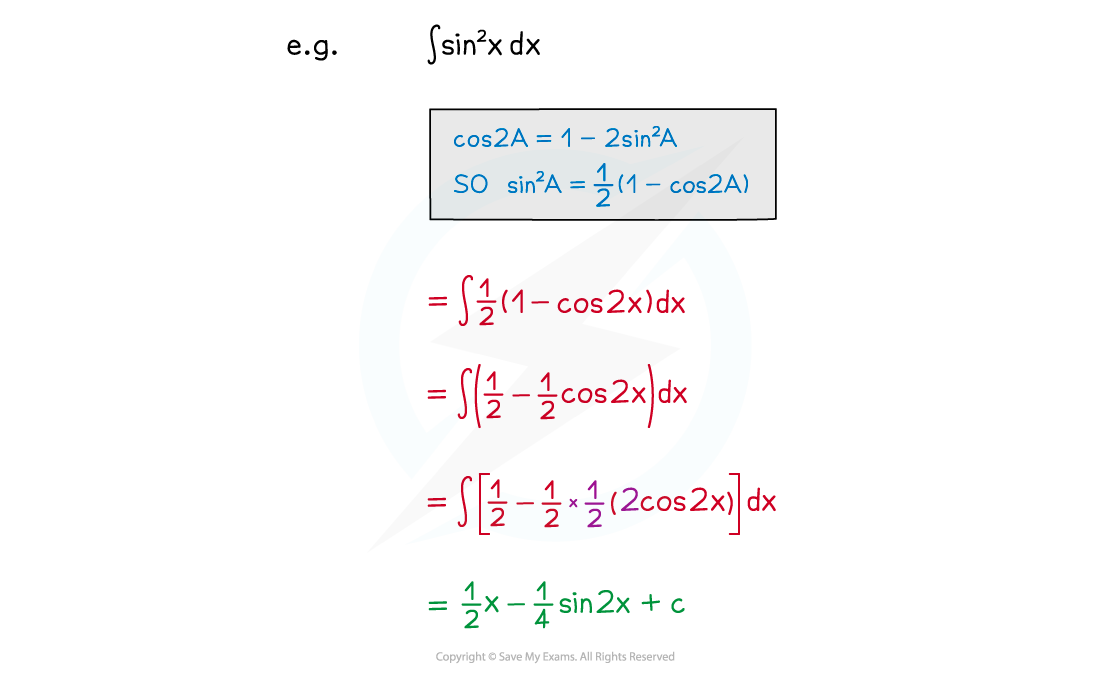#### How do I integrate tan?• This is a standard result from the formulae booklet

#### How do I integrate other trig functions?

• The formulae booklet lists many standard trigonometric derivatives and integrals
• Check both the “Differentiation” and “Integration” sections
• For integration using the "Differentiation" formulae, remember that the integral of f'(x) is f(x) !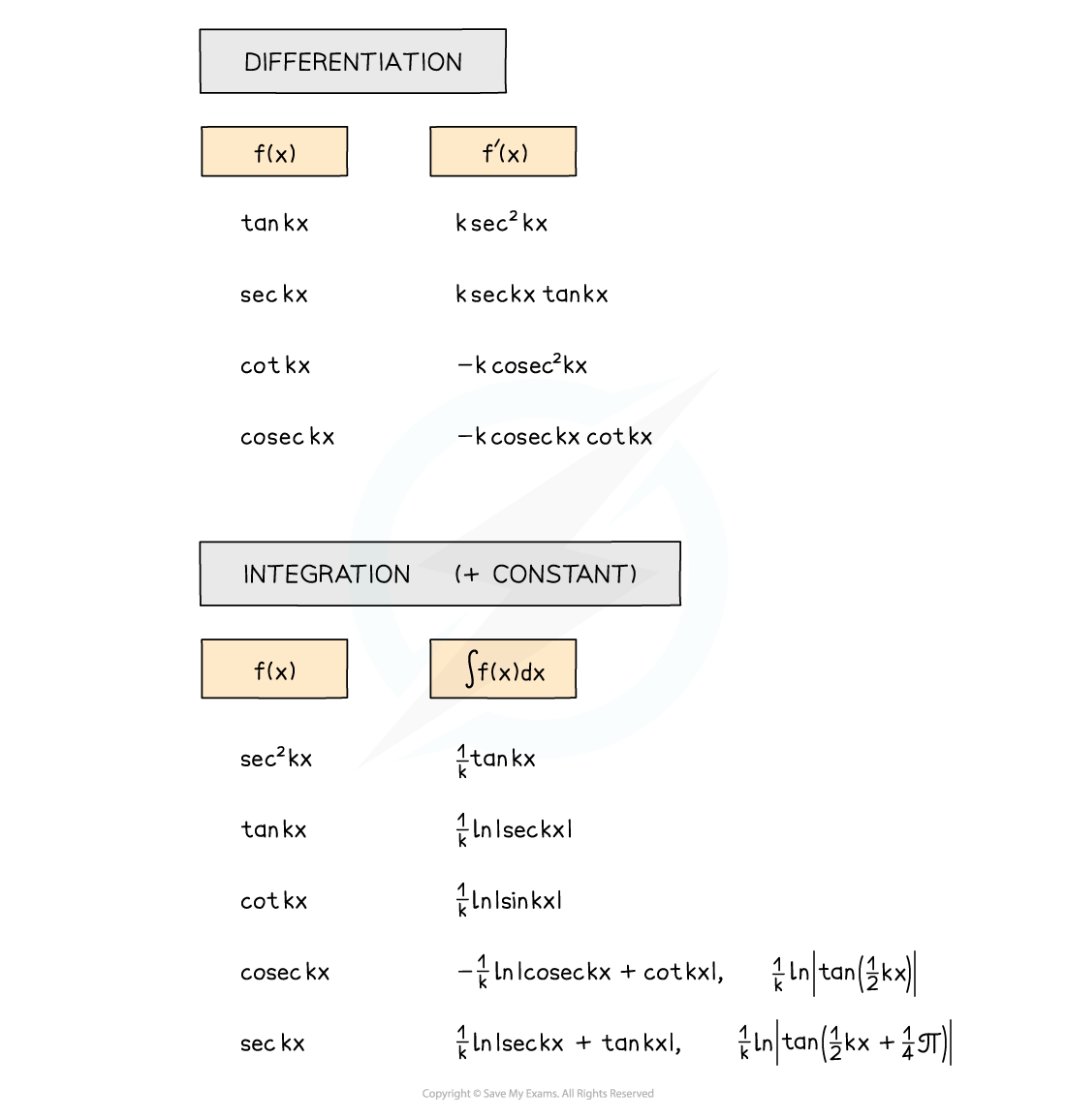• Experience, familiarity and recognition are important – practice, practice, practice!
• Problem-solving techniques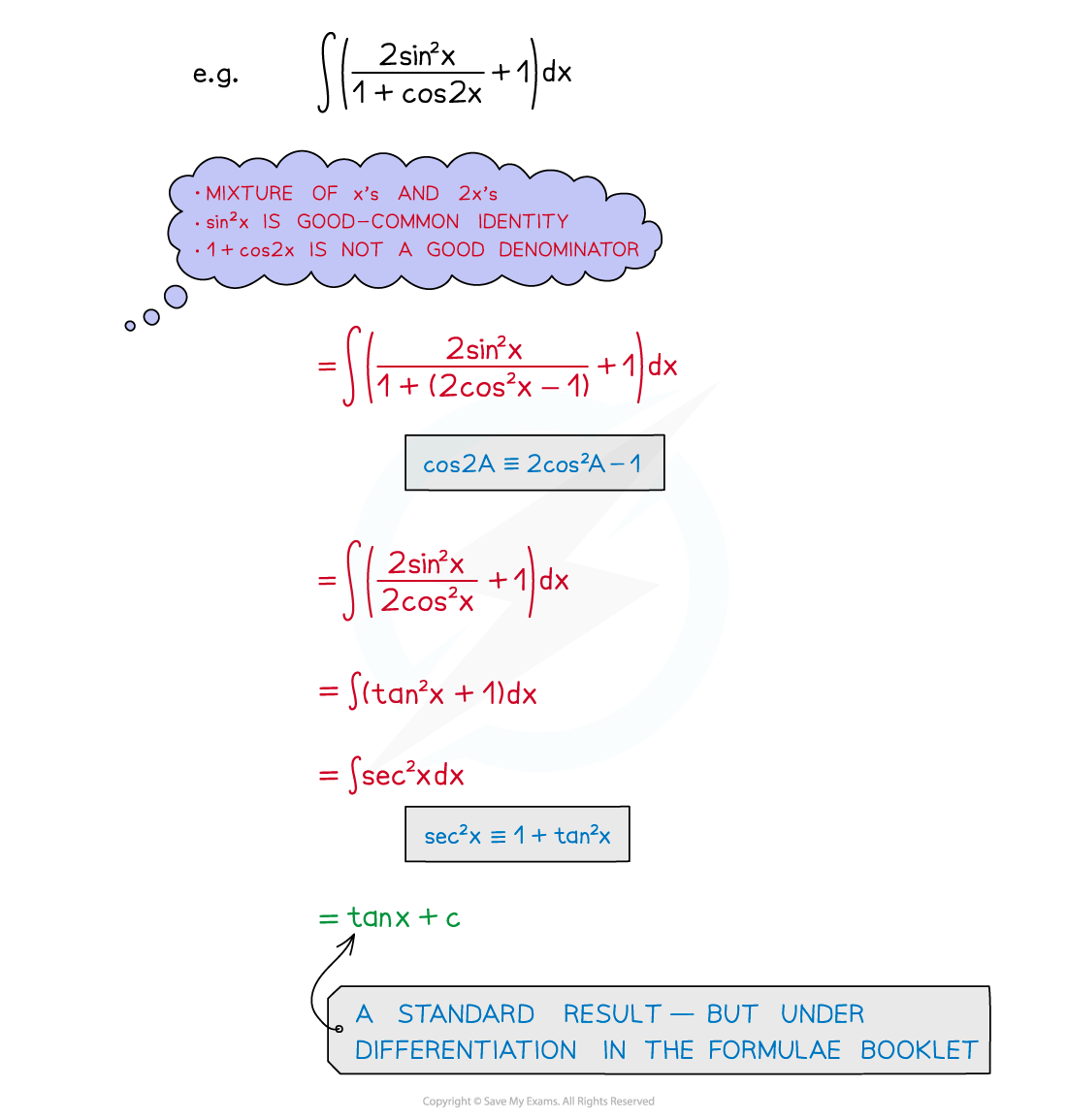#### Exam Tip

• Make sure you have a copy of the formulae booklet during revision.
• Questions are likely to be split into (at least) two parts:
• The first part may be to show or prove an identity
• The second part may be the integration
• If you cannot do the first part, use a given result to attempt the second part.

#### Worked Example